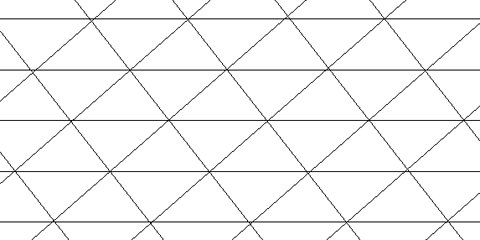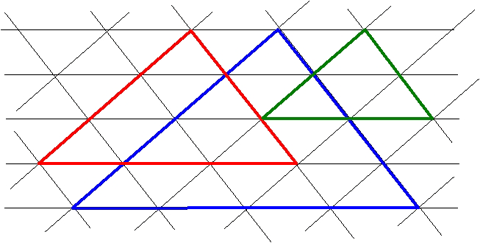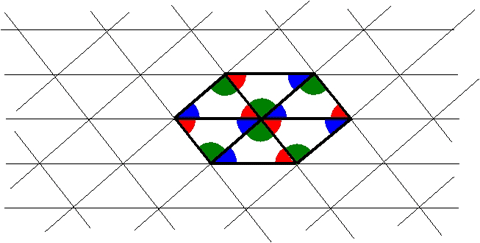Home > Patterns > Misunderstandings > Tessellations > Triangles tessellate

# Triangles tessellate

Here is a triangle tessellation.A triangle tessellation.

Look at the separate triangles. Half of the triangles are 'point up' and half are 'point down'. But they are all exactly the same size and shape — that is, they are congruent.

There are other geometrical concepts hidden in the tessellation.

• There are three sets of parallel lines, some horizontal, some sloping up to the right, and some sloping up to the left.
• There are several large triangles embedded in the diagram.
Each of these has the same shape as the small triangle, but their sides are two, three or four times as long and each triangle contains four, nine or sixteen small triangles.
The small and large triangles are similar.Similar triangles.

Six triangles fit around each 'point' of the tessellation.

At each point, there are six corners, consisting of two copies of each corner of the triangle — three on one side of a line and three on the other side.

In other words, the three corners of a triangle together make up a straight line.Six triangles fit around each point.

The triangle tessellation is a repeating pattern. Can you find a unit of repeat for the tessellation?